Mobile QR Code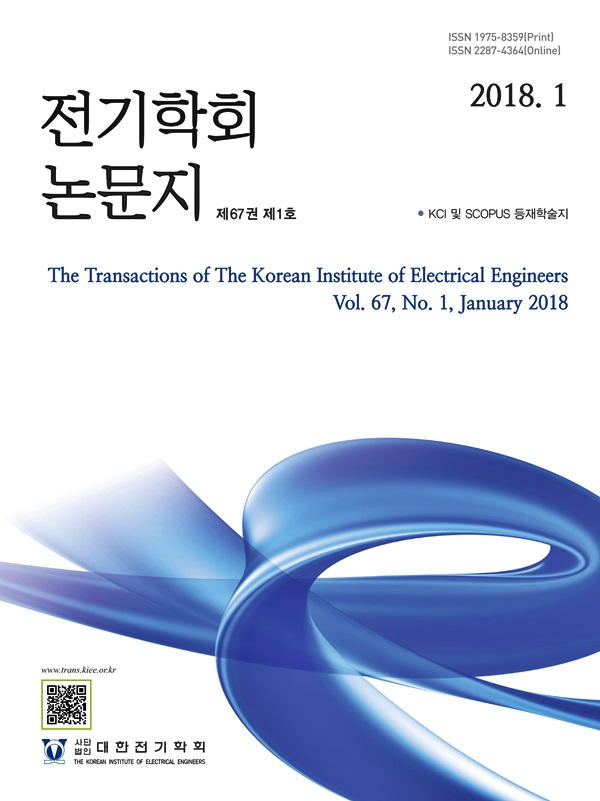#### The Transactions of the Korean Institute of Electrical Engineers

##### ISO Journal TitleTrans. Korean. Inst. Elect. Eng.
• SCOPUS
• KCI Accredited Journal

1. (Energy Innovation Industry R&D Dept. at Green Energy Institute.)
2. (Dept. of Electrical Engineering, Chosun University, Korea.)
3. (Wind·Ocean Power R&D Dept. at Green Energy Institute.)

Superconducting fault current limiter, Winding type, Magnetic field, Magnetic flux density, Superconducting wire

## 1. Introduction

With the recent increase in electric power demand, studies on renewable energy sources and connection to direct current (DC) systems that utilize distributed power sources have been actively conducted worldwide (1-3). DC systems have smaller losses than alternating current (AC) systems in terms of long-distance power transmission. Therefore, DC can compensate for insufficient power supply through connection to the Northeast Asian Super Grid high-voltage direct current (HVDC) because it is favorable for long-distance power transmission (4-5). For DC, however, the circuit breakers that block the fault current in the event of a fault are insufficient. In DC systems, it is difficult to block the fault current because there is no natural zero point, unlike in AC systems. In addition, DC generates high arcs in the blocking process, thereby causing secondary damage by fire and explosion (6). Therefore, for blocking systems to be used in HVDC systems, research must be conducted on arc extinction and safety in the blocking process, as well as on highly reliable blocking systems. As such, in this study, a superconducting DC blocking technology was proposed by combining the existing fault current limiting technology that uses superconductors with a DC circuit breaker. The use of superconductors can ensure reliability because the fault current is limited in a stable manner and the blocking operation is completed within a few milliseconds by the DC circuit breaker (7-8). The optimal winding type of the superconducting wire, which limits current, was derived in this study. For superconductors that limit the fault current, the current limitation is delayed as the inductance increases. Therefore, the blocking performances was compared and analyzed through the current limiting rate, quenching time of the superconductor, and the operation completion time of the DC circuit breaker.

## 2. Characteristics of each winding type

### 2.1 Theory

In terms of energy, the unit of work can be expressed in units such as calorific value. This can be confirmed by 식(1) and 식(2). The amount of work is determined by the relationship between the strength of the magnetic field and the magnetic flux density. Therefore, the amount of heat generated is proportional to the intensity and magnetic flux density of the magnetic field generated in the coil. In the case of superconductors, the closer the magnetic field strength is, the closer the critical field is. At this time, the impedance is generated as the superconductor transitions to the phase conduction state with the quench phenomenon. Depending on the winding types, the generation of magnetic field and the increase of magnetic flux density of the superconductor can induce fast quench characteristics. Therefore, in this paper, spiral and helical types, which are general winding methods, were designed using Maxwell program. The difference between the lengths of the superconductor and the magnetic field is generated by two winding types. As the quench speed of the superconductor is shortened, it is possible to secure the fast breaking characteristic of the DC circuit breaker that constrains and blocks the fault current.

##### (1)
$W_{s}=\dfrac{1}{2}\int_{v}H\bullet Bdv$ [J]

##### (2)
$Q_{s}\propto V_{s}I_{s}t$ [J]

$W_{s}$: magnetic energy of superconducting coil,

$H$ : magnetic field strength of superconducting coil,

$B$ : magnetic flux density of superconducting coil,

$Q_{s}$: calorific value,

$V_{s}$: voltage flowing in the superconducting coil,

$I_{s}$: current flowing in the superconducting coil

표 1. 각 선재 유형에 대한 설계 파라미터

Table 1. Design parameters for each wire type

 Type Spiral Helical Number of turns [turn] 4 5 Length [mm] 3 4 Height [mm] - 100 Innermost diameter [mm] 200 - Outermost diameter [mm] 250 Pitch [mm] 8 Thickness [mm] 1 Width [mm] 10 Inductance [mH] Calculated value 0.07 Measured value 0.07

### 2.2 Modeling and magnetic field distribution using the Maxwell program

표 1 shows the coil design of the superconducting current-limiting part. 그림 1(a) and (b) show the results of the analysis of each wire type’s magnetic field characteristics using Maxwell simulation. In Maxwell simulation, the magnetic flux density can be expressed as shown in 식 (3). The simulation analysis results showed that the magnetic flux density of the spiral wire type was 0.850 T, and its magnetic field intensity was 67.680$\times 10^{4}$ A/m. For the helical wire type, on the other hand, the magnetic flux density was 0.813 T, and the magnetic field intensity was 64.769$\times 10^{4}$ A/m. The spiral wire type had an approximately 0.037 T higher magnetic flux density and a 2.911$\times 10^{4}$ A/m higher magnetic field intensity. As mentioned earlier, increases in the magnetic flux density and magnetic field intensity can increase the blocking speed of the DC circuit breaker as they increase the calorific value generated during the quench of the superconductor. Finally, the inductance of the spiral wire type can be calculated using 식(4) and 식(5) while that of the helical type can be calculated using 식 (6) (9-10).

그림. 1. 보빈에 감긴 각 선재 종류 및 HTS 자기장 특성

Fig. 1. The characteristics of the magnetic field of each wire type and HTS wound on bobbin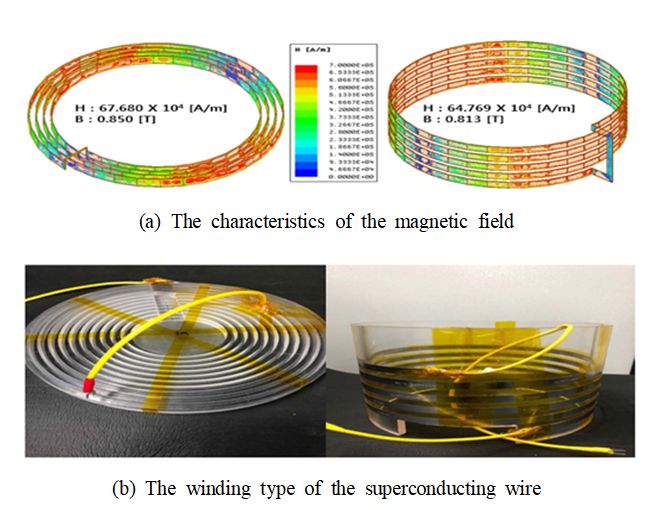##### (3)
$\dfrac{I ntegrate(Surface(Wire Rod),\: Mag(<B_{x},\:B_{y},\: B_{z}>))}{I ntegrate(Surface(Wire Rod),\:1)}$ $=B_{avg}$ [T]

##### (4)
$L_{spiral} =\dfrac{N^{2}A^{2}}{30A-11D_{i.n}}$ [mH]

##### (5)
$A=\dfrac{D_{i.n}+N(W+S)}{2}$

##### (6)
$L_{Helical}=\dfrac{D^{2}N^{2}}{0.45D+H}\times 10^{3}$ [mH]

$D_{i.n}$: Innermost diameter of the spiral coil,

W : Width of the superconductor,

S : Pitch of the superconducting wire,

N : Number of turns of the superconducting wire,

H : Height of the helical coil

### 2.3 Circuit design and parameters using PSCAD/ EMTDC

그림 2 shows the circuit diagram, which includes the superconducting current-limiting part that limits the fault current, and the mechanical DC circuit breaker that blocks the fault current, using PSCAD/EMTDC. The superconductor for limiting the fault current was designed using 식 (7) (11). In addition, an applied voltage of 100 kV and a steady-state current of 400 A were selected for the simulation, and the maximum fault current was set to 70 kA. As for the superconductor, (maximum impedance) was set to 5 Ω, and (quench time constant of the superconductor) was set to less than 2 ms. The L and C for generating resonance were 0.01 mH and 100 uF, respectively. Finally, the surge arrest (SA) for absorbing the residual current after blocking the fault current was set to 80 kV. Simulation analysis was conducted by selecting the same inductance of 0.007 mH for the two wire types.

그림. 2. PSCAD/EMTDC를 활용한 회로도 설계

Fig. 2. Design of the circuit diagram using PSCAD/EMTDC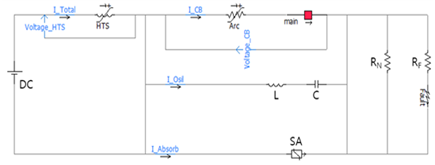##### (7)
$R_{SFCL}=\left\{\dfrac{0}{R_{m}\sqrt{1-\exp(-t/T_{SC})}}\right\}$

$R_{m}$: superconductor maximum shunt resistance,

$t_{SC}$: superconductor time constant

그림 3 shows the experimental results of each wire type that used the same inductance. The spiral wire type performed blocking approximately 0.0008 ms later than the helical wire type, but its blocking completion time of 0.133 ms was similar to that of the helical wire type. The resonance frequencies can be expressed using 식(8) and 식(9) through the oscillation of LC (12). Therefore, the helical wire type has a lower resonance frequency than the spiral wire type as the number of turns increases. As a result, the fault current by LC oscillation was increased by approximately 34 kA for the spiral wire type and by 28 kA for the helical wire type. If the length of the superconducting wire is analyzed instead of the same inductance, the inductance of the helical wire type increases. This further delays the blocking of the fault current.

##### (8)
$f=\dfrac{1}{2\pi\sqrt{LC}},\: L=\dfrac{n\Phi_{s}}{i}$ [J]

##### (9)
$f=\dfrac{1}{2\pi\sqrt{\dfrac{n\Phi_{s}}{i}}}$ [J]

그림. 3. PSCAD/EMTDC를 이용한 각 선재 유형에 따른 차단 특성

Fig. 3. The characteristics of the blocking for the each wire using PSCAD/EMTDC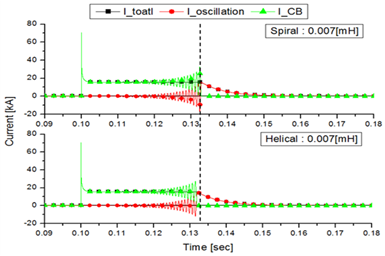## 3. DC blocking experiments for each wire type in the current-limiting part

### 3.1 Experimental conditions

Experiments for each wire type were carried out under the conditions shown in Tables 2 and 3. The configuration of the equipment for the experiment can be seen in 그림 4(a). Up to 80 batteries are connected in series to simulate a DC environment. In addition, the fault situation can be simulated using the accident generating device. The superconductor can maintain superconductivity by the cooling system, and the experiment was constructed so that the limited current can be cut off using a circuit breaker. Also, the circuit diagram configuration for the blocking test is shown in 그림 4(b). For the comparison of the two wire types, the applied voltage was increased from 400 to 550 V by 50 V increments. In addition, the normal load was set to 23.4 Ω, and the fault load was set to 1 Ω..

표 2. 각 유형에 대한 DC 차단 실험 조건

Table 2. The experimental conditions of the DC blocking for each wire type

 Battery [EA] Voltage [V] Fault current [A] Normal current [A] 32 400 449.78 17.09 36 450 486.75 19.23 40 500 523.71 21.36 44 550 560.67 23.50

표 3. 각 선재 타입에 대한 초전도 선재의 길이

Table 3. Length of the superconducting wire for each wire type

 Type Spiral Helical HTS Length [m] 3 4

그림. 4. DC 차단 실험 및 회로도

Fig. 4. DC blocking experiments equipment and circuit diagram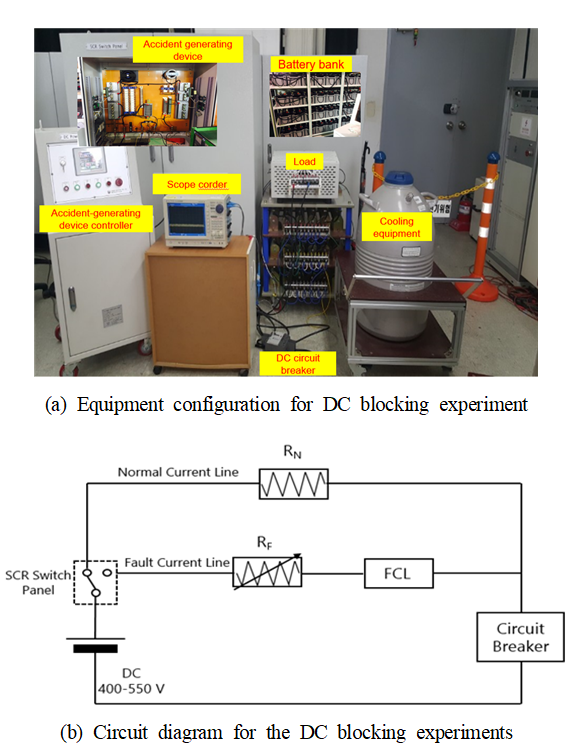그림. 5. 권선 종류에 따른 고장전류, 초전도체 저항, 차단 전류 특성

Fig. 5. The characteristics of the fault current, superconductor resistance and blocking current according to winding type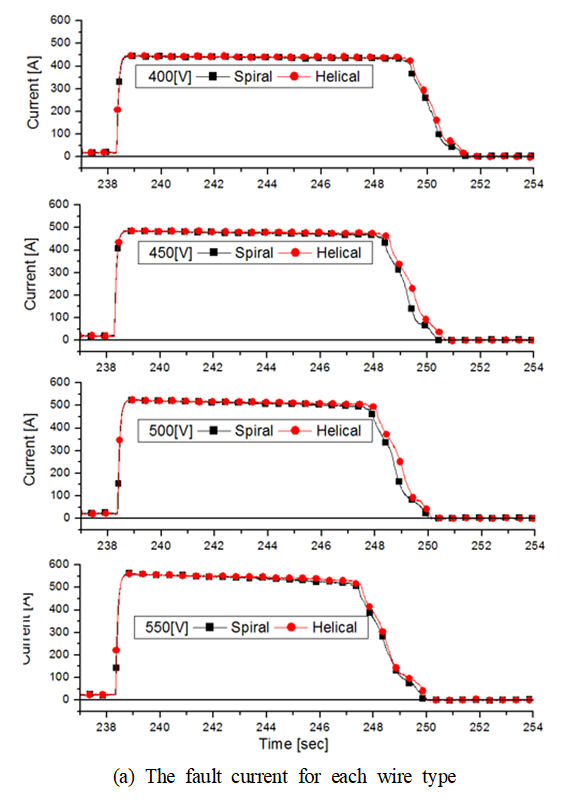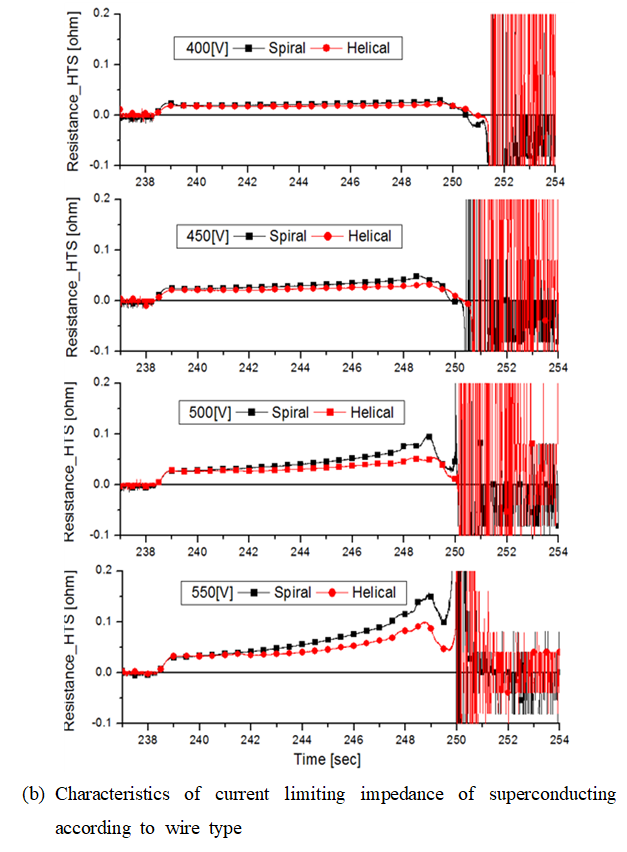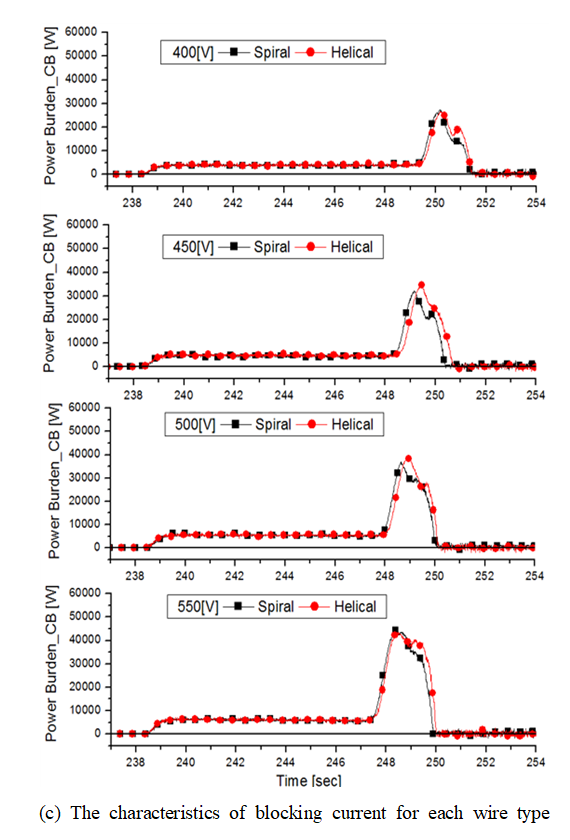### 3.2 Experimental results

그림 5(a) shows the current characteristics according to the voltage. As the voltage increased, the fault current limitation rates of the spiral wire type were found to be 9.39, 8.62, 8.52 and 11.63%. For the helical wire type, the fault current limitation rates were found to be 5.69, 5.24, 6.01 and 7.62%. 그림 5(b) shows the impedance characteristics according to the voltage. As the voltage increased, the impedance of the spiral wire type increased by 0.028, 0.045, 0.073 and 0.096 Ω. The impedance of the helical type, on the other hand, increased by 0.022, 0.028, 0.045, and 0.065 Ω. Based on the results shown in 그림 5(a) and (b), it was found that the fault current limitation ra tes of the spiral wire type were 3.70, 3.38, 2.51 and 4.01% higher than those of the helical wire type as the impedance of the spiral wire type was 0.006-0.031 Ω higher. Finally, the blocking time according to the voltage was analyzed using the power applied to the circuit breaker in 그림 5(c). The breaker opening times due to the introduction of the fault current were found to be 11.01, 10.11, 9.66 and 9.15 ms for the spiral wire type, and 11.13, 10.30, 9.81 and 9.24 ms for the helical wire type. The breaker opening time of the spiral wire type was approximately 0.09-0.19 ms faster than that of the helical wire type. Moreover, the breaker operation completion times were found to be 12.87, 12.01, 11.56 and 11.45 ms for the spiral wire type, and 12.95, 12.26 and 11.67 ms for the helical wire type. The spiral wire type exhibited approximately 0.06-0.25 ms faster blocking speed. 표 4 shows the experimental results of the spiral wire type, and 표 5 shows those of the helical wire type.

## 5. Conclusion

In this study, the characteristics of the current-limiting part for each wire type were analyzed to select the optimal structure of the superconducting DC circuit breaker for current limiting. First, the simulation results for each wire type were analyzed, and it was found that the application of the spiral wire type could improve the blocking speed by increasing the quench speed of the superconductor. Next, experiments were carried out using a small-scale experimental setup, and it was found that the spiral wire type exhibited up to 4.01 % higher fault current limitation rates. The spiral wire type also exhibited up to 0.19 ms faster breaker opening time and up to 0.25 ms faster breaker operation completion time. As superconductors are expensive, it is important to derive their maximum effect with the minimum quantity. Based on the simulation and experimental results in this study, it is expected that the application of the spiral wire type to the current-limiting part will improve the fault current limiting effect and increase the blocking speed of the circuit breaker.

### References

1
L. Chengyu, Z. Chengyong, X. Jianzhong, J. Yuke, Z. Fan, 2017, A Pole-to-Pole Short Circuit Fault Current Calculation Method for DC Grids, IEEE Trasn., Vol. 32, No. 6, pp. 4943-49532
Y. Wei, P. Rui, E. Joao, R. Francisco, Y. Li, Y. Chi, X. Tang, 2019, Analysis and Inspiration of the National Load all Powered by Renewable Energy in Portugal, IEEE Innovation Smart Grid. Technol(Asia)3
F. Z. Kadda, S. Zouggar, M. E. Hafyani, 2014, Contribution to the optimization of the electrical energy production from a Hybrid Renewable Energy system, 5th International Renewable Energy Congress(IREC)4
X. Jianzhong, Z. Sicheng, L. Chengyu, 2018, The Enhanced DC Fault Current Calculation Method of MMC-HVDC Grid With FCLs, IEEE Journal of Emerging and Selected Topics in Power Electronics, Vol. 7, No. 3, pp. 1758-17675
A. Kalair, N. Abas, 2016, Comparative study of HVAC and HVDC transmission systems, Renewable and Sustainable Energy Reviews, Vol. 59, No. , pp. 1653-16756
W. R. Leon Garcia, A. Bertinato, P. Tixador, B. Raison, 2016, Full-selective protection strategy for MTDC grids based on R-type superconducting FCLs and mechanical DC circuit breakers, 5th IET International Conference on Renewable Power Generation(RPG)7
H. W. Choi, H. S. Choi, I. S. Jeong, 2017, Characteristics Analysis of Superconductivity DC Interrupting System in the Grid-Connected PV System with EMTDC/ PSCAD, 16th International Superconductive Electronics Conference(ISEC)8
H. S. Gu, S. Y. Park, 2019, Failure Characteristics Analysis of DC Circuit Breaker According to Type of Current Limiting Unit Using Superconductor, Korean Institue. Electrical Engineer, Vol. 68, No. 129
S. S. Mohan, M. M. Hershenson, S. P. Boyd, 1999, Simple accurate expressions for planar spiral inductances, IEEE Journal of Solid-State Circuits, Vol. 34, No. 10, pp. 1419-142410
J. W. Kim, H. H. Ji, Y. G. Choi, Y. H. Yun, K. H. Kim, 2010, Study on Arrangement of Self-Resonant Coils in Wireless Power Transfer System Based on Magnetic Resonance, The Journal of Korean Institute of Electromagnetic Engineering and Science, Vol. 21, No. 6, pp. 564-57211
H. Y. Choi, I. S. Jeong, S. Y. Park, 2018, Characteristics of Superconducting Coil-Type DC Fault Current Limiter to Increase Stability in the Grid Connection PV Generation System, IEEE Transactions on Applied Superconductivity, Vol. 28, No. 3, pp. 560090412
I. S. Jeong, H. Y. Choi, 2018, Analysis of S-Parameter Using Different Materials for the WPT Resonance Coil, IEEE Transactions on Applied Superconductivity, Vol. 28, No. 3, pp. 0500405## 저자소개

Graduated from the Department of Mechatronics Engineering, Chosun University in 2018, Graated from the same graduate school in 2020 (Master of Engineering).

Currently Researcher of Energy Innovation Industry R&D Dept. at Green Energy Institute.

Graduated from the Department of Electrical Engineering, Chosun University in 2016.

Graated from the same graduate school in 2018 (Master of Engineering). 2018-present: graduate school (complete a doctorate)

Graduated from the Department of Electrical Engineering, Chosun University in 2012.